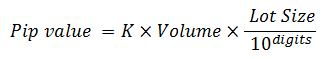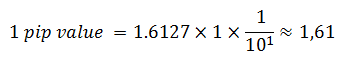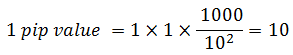# CFD - Pip value

The pip value for CFDs  is calculated using the following formula:where:

• K — Profit Currency (second currency in the symbol) to Deposit Currency conversion rate.
• Volume — volume of position in lots.
• Lot Size — number of ounces, barrels or commodity units in one lot.
• Digits — number of decimal places.

Example:

• Conversion rate (K): 1.6127
• Volume: 1  lot
• Lot Size: 1
• Digits: 1Pip value: 1.61  (USD)

Example: